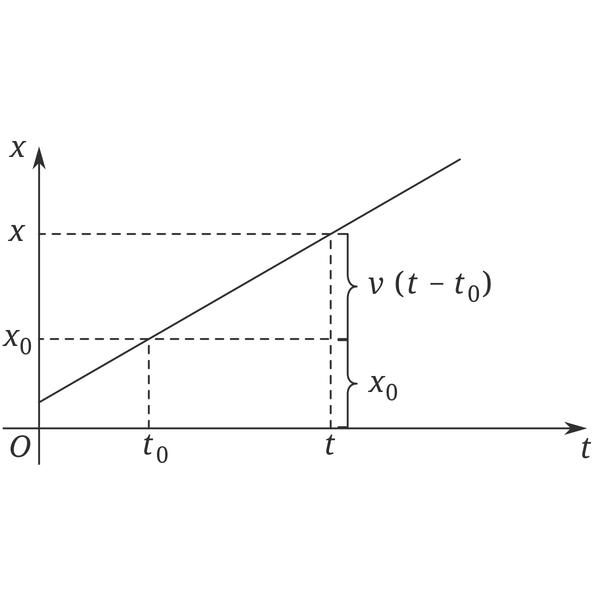# Uniform linear motion

## Velocity

Uniform linear motion (5 photos)

For objects that do uniform linear motion, in different displacements or time periods, the displacement and The ratio of time is a fixed value, and this fixed value is the speed of the movement. (Note: The speed is a fixed value, which means that the speed and the direction of the movement are the same, because the speed is a vector, there is a size and a direction.) The size of the speed directly reflects the speed of the object's movement. In a uniform linear motion, the average speed and the instantaneous speed are equal, and the magnitude of the average speed and the average speed are also equal.

## Acceleration

1. The acceleration of an object moving at a constant speed is zero.

2. The combination of ideal state and reality: uniform linear motion is not common, because the condition for an object to perform uniform linear motion is that it is not subject to external force or the sum of external forces is zero, but we can approximate some motions The ground is regarded as a uniform linear motion. For example: a section of the skate after the skater stops exerting force, the movement of customers standing on the escalator in a shopping mall, and so on. We can use the formula v=s/t to find their movement speed. In the formula, s is displacement, v is speed and constant vector ,t is the time taken for displacement s. It can be seen from the formula that displacement is a proportional function of time: displacement is directly proportional to time.3. When the object is moving in a straight line at a uniform speed, the force is balanced.

4. When an object performs mechanical motion, it can be divided into linear motion and curved motion according to the curve of the motion route. In linear motion, according to whether the speed changes, it is divided into uniform linear motion and variable linear motion.

## Points to note

(1) Speed ​​is a physical quantity that expresses the speed of movement of an object, and speed can be represented by the symbol V. In the International System of Units (SI), the main unit of speed is m/s, which is pronounced: meters per second. Commonly used units are km/h, m/min and so on.

(2) The speed of an object moving in a straight line at a constant speed remains unchanged. Therefore, if you know the speed of movement at a certain moment (or a certain distance), you will know that it is in any period of time. Speed ​​within or at any moving point.

(3) When an object is subjected to two or more forces, if it can remain stationary or move in a straight line at a uniform speed, we say that the object is in a state of equilibrium.

(4) From a mathematical point of view, the formula s=vt cannot be understood as that the speed of the object's movement is proportional to the distance and inversely proportional to the time. The characteristic of uniform linear motion is that the magnitude and direction of the instantaneous speed remain unchanged, and the acceleration is zero, which is an idealized motion.

(5) When a charged particle moves under the combined action of constant force and Lorentz force, as long as it moves in a straight line, it must move in a straight line at a uniform speed. (Reason: A force like F Luo will change with the change of speed, that is, the speed directly affects the resultant force, and the resultant force directly affects the acceleration, that is, the direction of movement.)

(6) Uniform linear motion is only Ideal state.

Related Articles